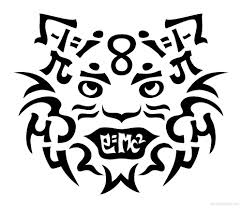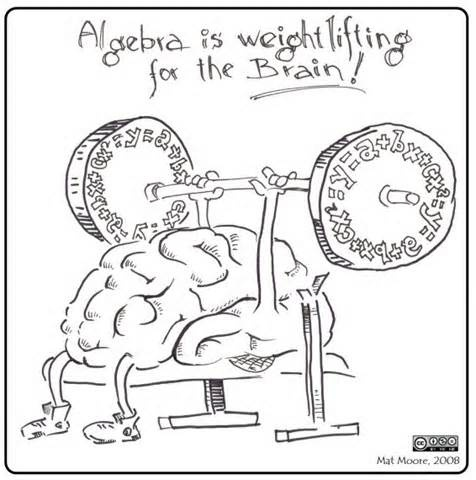• Select a School
Select a School

## Mathematics

“Education is not the learning of facts, but the training of the mind to think.”

-Albert EinsteinWelcome to Mathematics

with

Mr. Savatt

Overview

The math curriculum for Fundamentals of Algebra consists of the following topics

 Real Numbers Scientific Notation Functions Volume Irrational Numbers Proportional Relationships Linear Relationships Scatterplots Exponential Expressions Linear Equations Object Transformations Two-Way Tables Cube and Square Roots Systems of Equations Pythagorean Theorem Linear/Non-Linear Models

The Curriculum for Honors Geometry will consist of:

 points, lines planes and angles deductive reasoning parallel lines and planes congruent triangles quadrilaterals inequalities in geometry similar polygons right triangles circles areas of plane figures areas and volumes of solids coordinate geometry

Supplies

All students are expected to bring the following every day:

Charged Chromebook

Textbook

Notebook

Pencil

Homework

tbd

Contact Me!

Email:   [email protected]

Phone: 717-244-4022 ext.